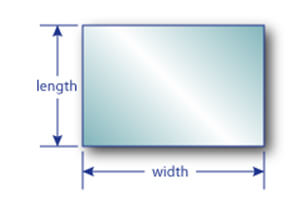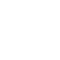Glass Calculate
To determine your glass' weight in LBS.* just enter your glass shape, dimensions, and glass thickness into the glass weight load calculator then click on the calculate button.
* Weight calculations are approximate
1. Select Shape
2. Enter DimensionsInches Fraction of an Inch
All measurements are in inches
Width:
Height:Inches Fraction of an Inch
All measurements are in inches
Width:
Height:Inches Fraction of an Inch
All measurements are in inches
Diameter:Inches Fraction of an Inch
All measurements are in inches
Width:
Height:
3. Choose Thickness
4. Calculate
Calculate Glass Weight
Figures are approximate – Dulles Glass and Mirror does not guarantee accuracy and is not responsible for any miscalculations.
How do I figure glass weight per square foot of glass?
Multiply 1.7 pounds for every 1/8" square foot of glass. For example, a 3/8" panel of glass that is 3 x 6 would be 1.7 x 3 (from 3/8") x 3 x 6 = 91.8 pounds.
Here are common, approximate weights for glass per square feet:

SS - 1.20 DS - 1.60 5/32 – 2.00 3/16 – 2.45 1/4 - 3.00 3/8 – 5.00 0-1/2- 6.50 5.8 – 8.00 3/4 – 9.97 IG unit – 2 x glass

For square and rectangular glass, square footage is determined by multiplying the width x the length of the table and dividing the answer by 144. You will need two measurements of the glass. Use the tape measure to measure the length and the width of the glass in inches. Next, multiply the two measurements of the glass. Divide the answer by 144. This will give you the square footage of the glass.
To determine the square footage of a round glass, measure the diameter of the glass using the tape measure (the diameter is the length across the glass directly through the center). Divide the diameter in half. The resulting number is the "radius". Convert the radius measurement to decimal by dividing the inches portion of the measurement by 12 (for example, a glass with a radius of 3 feet 9 inches has a decimal radius of 3.75 feet).
Now, calculate the area of the glass using the formula for the area of a circle: Area equals pi times the radius squared. Pi is a constant value of 3.1416. To square is to multiply by the number by itself. In the example, the round glass calculation will be 3.1416 x 3.75 x 3.75 equals 44.18 square feet, rounded to two places.
The area of an oval glass can be calculated by thinking of the glass as a rectangle with half of a round glass on each end. Do the calculations for the rectangle and round parts and add them together.
Here are the decimal vs. fraction equivalents:

1/16 - .0625 1/8 - .125 3/16 - .1875 1/4 - .250 5/16 - .3125 3/8 - .375 7/16 - .4375 1/2 - .500 9/16 - .5625 5/8 - .625 11/16 - .6875 3/4 - .750 13/16 - .8125 7/8 - .875 15/16 - .9375 1 - 1.000•